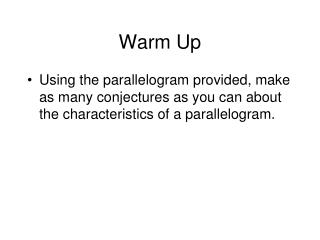DownloadDownload PresentationWarm Up

# Warm Up

Download Presentation## Warm Up

- - - - - - - - - - - - - - - - - - - - - - - - - - - E N D - - - - - - - - - - - - - - - - - - - - - - - - - - -
##### Presentation Transcript

1. Warm Up • Using the parallelogram provided, make as many conjectures as you can about the characteristics of a parallelogram.

2. Answers to homework pg 7 in packet

3. Chapter 6.2: Properties of Parallelograms Students will apply the properties of parallelograms

4. Definition of a Parallelogram • a quadrilateral in which both pairs of opposite sides are parallel

5. Given: ABCD is a parallelogram.Prove: A B D C • Statements Reasons • ABCD is a 1. Given • Draw BD 2. Through any two points there exists exactly one line • AB || CD, AD || CB 3. Definition of parallelogram • ∠ABD ≅ ∠CDB, 4. Alternate Interior Angles • ∠ADB ≅ ∠CBD • DB≅DB 5. Reflexive Property of Congruence • ∆ADB ≅ ∆CBD 6. ASA Congruence Postulate • AB ≅ CD, AD ≅ CB 7. CPCTC

6. A B Theorem 6.2 If a quadrilateral is a parallelogram, then its opposite sides are congruent. Theorem 6.3 If a quadrilateral is a parallelogram, then its opposite angles are congruent. Theorem 6.4 If a quadrilateral is a parallelogram, then its consecutive angles are supplementary. Theorem 6.5 If a quadrilateral is a parallelogram, then its diagonals bisect each other. D C

7. Practice Problems Each figure is a parallelogram. • VF = 36 cm, 2. What is the perimeter? EF = 24 cm, EI = 42 cm x + 3 x - 3 17 E V N F I What is the perimeter of NVI?

8. Let’s Review… In a parallelogram: • opposite sides are congruent • opposite angles are congruent • consecutive angles are supplementary • diagonals bisect each other